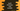# Swift dictionary map explanation with example## Introduction :

Swift dictionary provides one map method for mapping a function to all of its elements. This method is defined as below :

``func map<T>(_ transform: ((key: Key, value: Value)) throws -> T) rethrows -> [T]``

It takes one parameter transform, a closure that is applied to each of its elements. This closure accepts key and value and returns the new converted value.

This function returns one array. Not a dictionary. If you want a dictionary, you need to convert that array to a dictionary.

## Example of dictionary map :

Let’s consider the below example :

``````var my_dict = ["one" : 1, "two": 2, "three": 3]

var dict_array = my_dict.map{key,value in (key.uppercased(), value*2)}

print(dict_array)``````

Here, my_dict is the given dictionary with string keys and integer values. The map method mapped the elements and created the array dict_array. It prints the below output :

``[("ONE", 2), ("TWO", 4), ("THREE", 6)]``

## Dictionary map - convert the array to a dictionary :

This is an array. To create a dictionary, we can use the init(uniqueKeysWithValues: S) method :

``````var my_dict = ["one" : 1, "two": 2, "three": 3]

var dict_array = my_dict.map{key,value in (key.uppercased(), value*2)}

var new_dict = Dictionary(uniqueKeysWithValues: dict_array)

print(new_dict)``````

It will print :

``["ONE": 2, "THREE": 6, "TWO": 4]``

## Change the type of key and value :

We can also change the type of all keys and values using the closure :

``````var my_dict = ["one" : 1, "two": 2, "three": 3]

var dict_array = my_dict.map{key,value in (value, key)}

var new_dict = Dictionary(uniqueKeysWithValues: dict_array)

print(new_dict)``````

Here, we have changed the value to key and key to value.

It will print :

``[1: "one", 2: "two", 3: "three"]``

Official doc